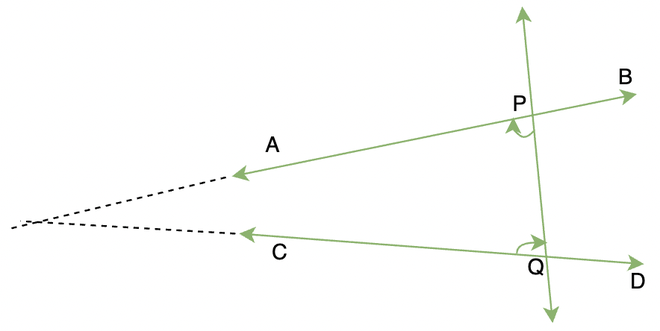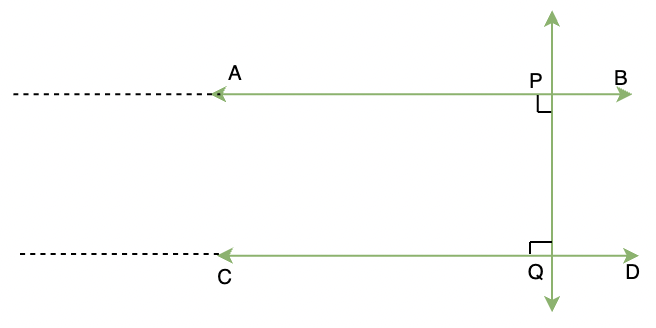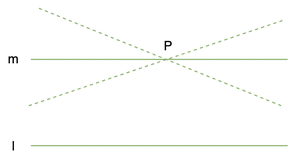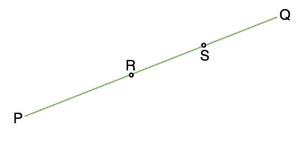Open in App
Not now

# Equivalent Version of Euclid’s Fifth Postulate

• Last Updated : 18 Mar, 2021

Geometry has originated from a variety of civilizations. Almost every major civilization has studied and used geometry in its prime. Egyptian and Indian civilizations were more focused on using geometry as a tool. Euclid came and changed the way people used to think in geometry. Instead of making it a tool, he thought of geometry as an abstract model of the world in which he lived in.

### Euclidean Geometry

According to Euclid,

“A solid has shape, size, position, and can be moved from one place to another. Its boundaries are called surfaces. They separate one part of the space from another and are said to have no thickness. The boundaries of the surfaces are curved or straight lines. These lines end in points.”

Starting from these definitions, he assumed certain properties which were not to be proved. They were termed as “Obvious Universal Truth”.  He divided them into two types,

1. Postulate: They are assumptions specific to geometry.
2. Axiom: They are usually common notions.

Let’s quickly look at the axioms of Euclid.

1. Things which are equal to the same thing are equal to one another.
2. If equals are added to equals, the wholes are equal.
3. If equals are subtracted from equals, the remainders are equal.
4. Things which coincide with one another are equal to one another.
5. The whole is greater than the part.
6. Things which are double of the same things are equal to one another.
7. Things which are halves of the same things are equal to one another.

Most of these axioms are self-explanatory.

### Postulates of Euclid

Postulate 1: A straight line may be drawn from any point to any other point.

Postulate 2: Given two distinct points, there is a unique line that passes through them.

Postulate 2: A terminated line can be produced indefinitely.

Postulate 3: A circle can be drawn with any centre and any radius.

Postulate 4: All right angles are equal to one another.

Postulate 5: If a straight line falling on two straight lines makes the interior angles on the same side of it taken together less than two right angles, then the two straight lines, if produced indefinitely, meet on that side on which the sum of angles is less than two right angles.

Most of these postulates are pretty simple and straightforward to think about except the postulate 5. Let’s look at its explanations and its equivalent versions in detail.

### Equivalent Versions of Fifth Postulate

The fifth postulate states that,

“If a straight line falling on two straight lines makes the interior angles on the same side of it taken together less than two right angles, then the two straight lines, if produced indefinitely, meet on that side on which the sum of angles is less than two right angles.”

This postulate holds a significant place in the history of mathematics.We can see in the figure that both the interior angles are less than 90°. So, their sum must be less than 180°. So, when they are extended they intersect at a particular point. This won’t happen if the internal angles are 90° or greater than 90°There are more than one equivalent versions of this postulate, one of them is called Playfair’s Axiom.

### Playfair’s Axiom

“For every line “l” and for every point “P” lying not on “l”, there exists a unique line “m” passing through P and parallel to “l”.From the above figure, we can see that there are multiple lines that pass through P but there is only one single line that passes through it and is parallel to “l”.

We can also rewrite this statement in another form,

“Two distinct intersecting lines cannot be parallel to the same line.”

Note (Fact): Euclid did not require the fifth postulate to prove his first 28 theorems. Many mathematicians including Euclid were convinced that the fifth postulate is actually a theorem that can not be proved. Several attempts were made but no one was able to prove the fifth postulate.

### Sample Problems

Let’s see some examples regarding these postulates and axioms,

Question 1: Harsh’s salary equal to Ram’s salary. Due to the Covid-19 recession, Harsh and Ram’s salaries are made half. The final salary of Ram will still be equal to Harsh. This is as per

1. 1st Axiom.
2. 7th Axiom.
3. 6th Axiom.
4. 2nd Axiom.

7th Axiom states that,

Things which are halves of the same things are equal to one another.”

This axiom can be applied here directly. Thus, the answer is (2).

Question 2: Boundaries of Solids are:

1. Lines
2. Points
3. Surface
4. Curves

According to Euclid’s definition,

“A solid has shape, size, position, and can be moved from one place to another. Its boundaries are called surfaces. They separate one part of the space from another, and are said to have no thickness. The boundaries of the surfaces are curves or straight lines. These lines end in points.”

This definition states that boundaries of solids are called surfaces.

Question 3: In the figure given below, the line-segment has PS = RQ. Prove that PR = SQ.Given that PS = RQ

From the figure we can say,

PS = PR + RS

QR = RS + SQ

From the Euclid axiom number 3 stated above, we know that

“If equals are subtracted from equals, the remainders are also equal.”

Since, PS = QR

Subtracting RS from both sides.

⇒ PS – RS = QR – RS

⇒ PR = QS

Question 4: It is known that a + b = 18, and a = c. Prove that c + b = 18.

It is known by the Euclid axioms studied above,

“If equals are added to equals, the wholes are equal.”

The given equations are,

a + b = 18 ….. (1),

And a = c….. (2)

From the equations (1) and (2), we get.

a + b = c + b ….(3)

Now from equation (1) and equation (3).

We can conclude that

b + c = 18.

Question 5: It is known a + b = 11, then a + b + c = 11 + c. The Euclid axioms that illustrates this statement is,

1. 1st axiom.
2. 3rd axiom
3. 4th axiom
4. 2nd axiom